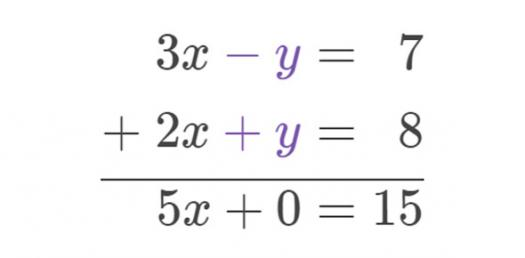# Algebra Quiz Chapter 4

17 Questions | Attempts: 424
ShareSettingsWhen it comes to graphs one has to know which the dependent is and independent variable and the different graphs that exist. Do you want to test out your algebra skills when it comes to graphs and interpreting them? Take up the quiz below and test yourself out. All the best!

• 1.
Which situation would be represented by a graph with points that are not connected?
• A.

The temperature of soup as it cools to room temperature

• B.

The height of a plant as it grows

• C.

The distance traveled on a bike

• D.

The number of shoppers who visited a store each day of the week

• 2.
Which relation is expressed by the mapping diagram below?
• A.

{(3,-1), (6,4), (8,4)}

• B.

{(-1,3), (6,4), (4,8)}

• C.

{(8,4), (6,4), (4,-1)}

• D.

{(4,3), (6,-1), (8,4)}

• 3.
What is the domain of the relation below? (assume that all inequalities in the answers are inclusive)
• A.

-3 < x < 3

• B.

-4 > x > 2

• C.

4 < x < -2

• D.

-4 < x < 2

• 4.
What is the range of the relation below? x 3 6 8 9 y 0 5 6 7
• A.

{3, 6, 8, 9}

• B.

{0, 5, 6, 7}

• C.

{0, 6, 8, 9}

• D.

{3, 6, 6, 9}

• 5.
Which of the following relations is a function?
• A.

{(1,-6), (3,-5), (1,0)}

• B.

{(0,5), (5,-1), (5,9)}

• C.

{(6,1), (6,2), (6,3)}

• D.

{(0,8), (1,7), (2,6)}

• 6.
Which equation shows the relationship between x and y in {(1, -3), (2, -1), (3, 1)}?
• A.

Y = x - 4

• B.

Y = 2x - 5

• C.

Y = 2x

• D.

Y = x – 2

• 7.
Which function could represent the following situation: “An internet café charges \$0.20 per minute.”
• A.

F(m) = m + 0.20

• B.

F(m) = m/20

• C.

F(m) = 0.20m

• D.

F(m) = 20m

• 8.
An architect must convert 216, 183, and 129 yards to feet. Which function rule describes the situation?
• A.

F(y) = y/3

• B.

F(y) = 3y

• C.

F(y) = 3/y

• D.

F(y) = y – 3

• 9.
Evaluate the function  when x = -6.
• A.

-14

• B.

-6

• C.

2

• D.

10

• 10.
Which is the dependent variable in the following situation? “The teacher ordered two binders for each student.”
• A.

Total cost

• B.

Date of order

• C.

Number of binders

• D.

Number of students

• 11.
Find the next three terms of the arithmetic sequence: 0, -1.5, -3, -4.5, …
• A.

-6.5, -8, -9.5

• B.

-6, -7.5, -9

• C.

-5, -6.5, -7

• D.

-4, -5.5, -6

• 12.
What is the 18th term of the arithmetic sequence 2, -2, -6, -10, ….
• A.

-72

• B.

-70

• C.

-68

• D.

-66

• 13.
Which function is graphed for the domain {-2, 0, 2, 4}?
• A.

Y = x - 1

• B.

Y = │x│- 1

• C.

Y = 2x + 6

• D.

Y = │x - 1│

• 14.
Which is the dependent variable in the following situation? ¨Milke sells for \$3.39 per gallon.¨
• A.

Number of gallons

• B.

Total cost

• C.

Expiration date

• D.

Time of purchase

• 15.
Which of the following is NOT a function?
• A.

{(1, 5), (3, -1), (7, 9)}

• B.

{(1, 5), (2, 5)}

• C.

{(1, 1), (2, 2) (3, 3)}

• D.

{(5, 1), (5, 2)}

• 16.
Which relation could this graph represent?
• A.

{(1, 3.5), (2.5, 2), (3, 2)}

• B.

{(1, 4.5), (3.5, 2), (3, 2)}

• C.

{(3.5, 1), (2, 2.5), (2, 3)}

• D.

{(4.5, 1), (2, 3.5), (2, 3)}

• 17.
Evaluate the function when x = -11.
• A.

-358

• B.

-38

• C.

38

• D.

358

## Related TopicsBack to top
×

Wait!
Here's an interesting quiz for you.